### Home > A2C > Chapter Ch10 > Lesson 10.2.3 > Problem10-95

10-95.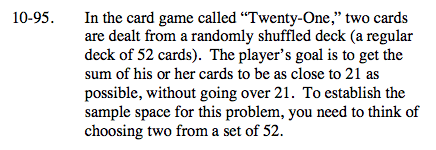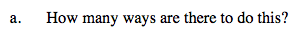$_{n}C_{r}=\frac{n!}{r!(n-r!)}$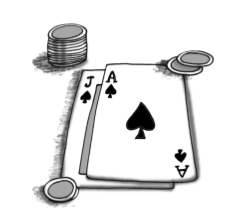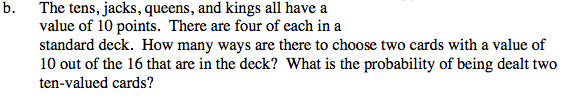How many ways are there to select two cards from the set of ten-valued cards?

Divide this number by the total number of 2-card hands.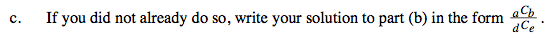$\frac{_{2}C_{1}6}{_{2}C_{5}2}$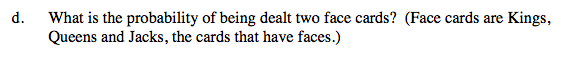See part (b).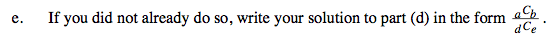See part (c).